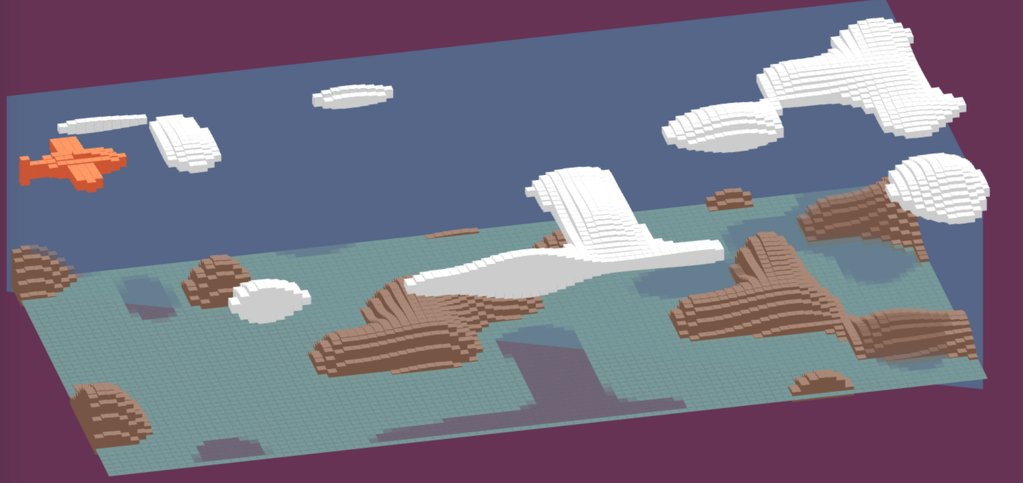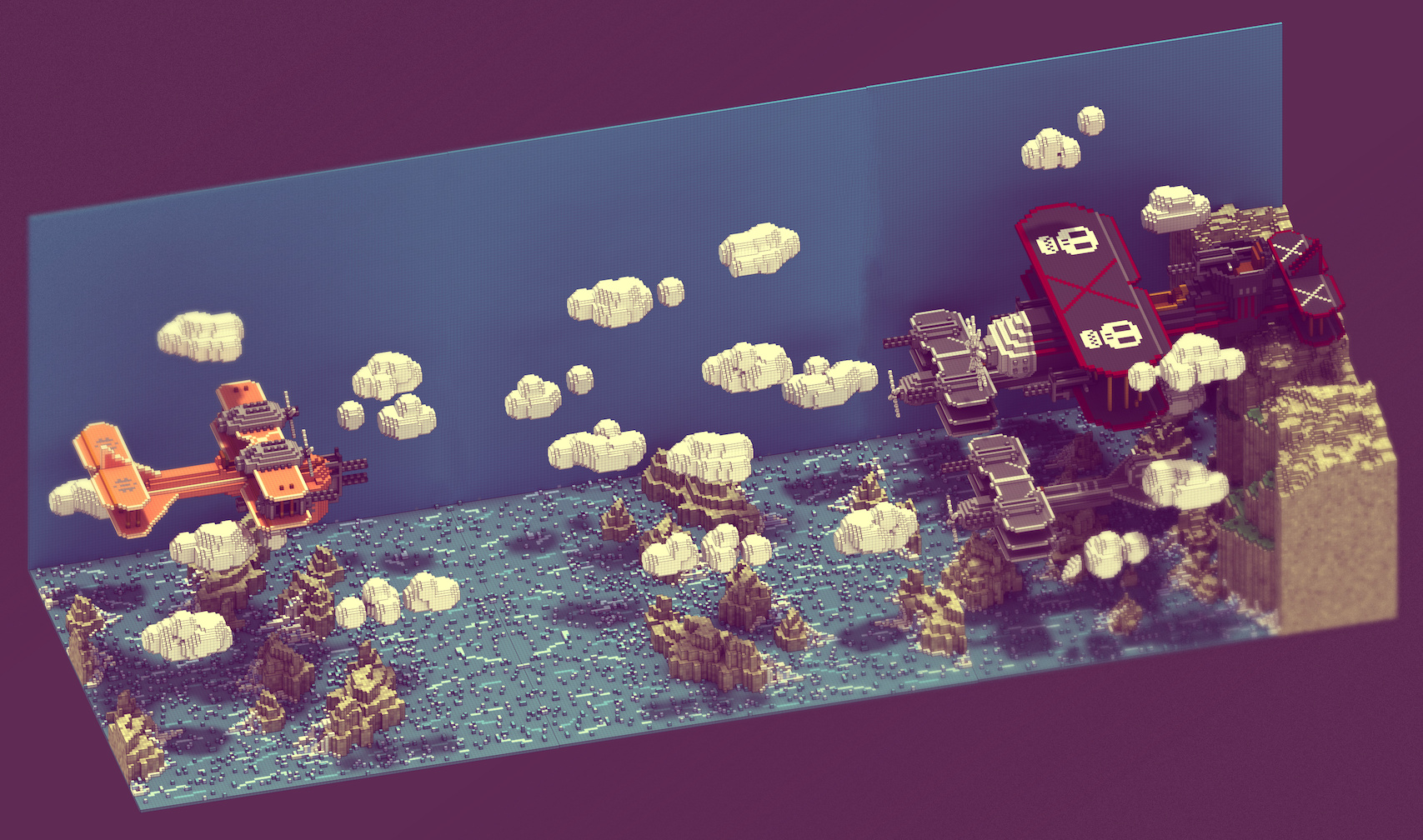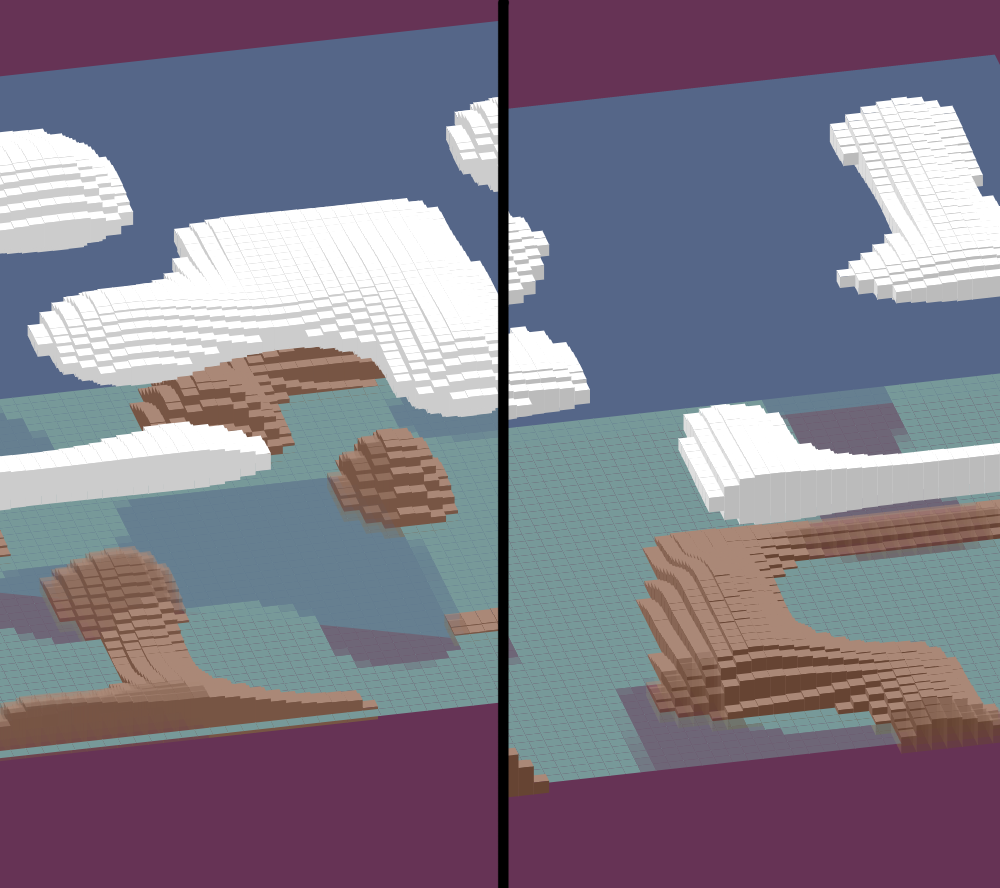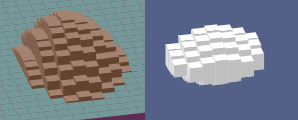# Voxeling – js1k 2016 postmortem

## blogThis year, as usual, I got the inspiration from somewhere else 🙂
Checking twitter while commuting I saw a re-tweet with a voxel image, I dug up a bit and, to be honest, I was really impressed by the amazing work of @Sir_carma:
More work of Sir_carma
More specifically in this voxel image:So I did my best to recreate it in 1k and here is the result:
http://js1k.com/2016-elemental/demo/2497
or go to the js1k page for bigger preview: http://js1k.com/2016-elemental/demo/2497
and, of course, do not hesitate to check all the other amazing entries this year: http://js1k.com/2016-elemental/demos

Some details of the source code, scroll down to see the whole thing:
First I created a height map of the plane, but to optmize it a bit, I made it a bit smaller & symmetric:

``````//full plane
p = [0, 0, 0, 0, 0, 0, 0, 1, 0, 0, 0, 0, 0,
0, 0, 0, 0, 0, 0, 1, 1, 1, 0, 0, 0, 0,
0, 0, 0, 0, 0, 0, 1, 1, 1, 0, 0, 0, 0,
0, 0, 0, 0, 0, 0, 1, 1, 1, 0, 0, 0, 0,
0, 0, 0, 0, 0, 0, 1, 1, 1, 0, 0, 0, 0,
0, 0, 0, 0, 0, 0, 1, 0, 1, 0, 0, 0, 0,
1, 0, 0, 0, 0, 0, 1, 1, 1, 0, 0, 0, 0,
1, 1, 0, 0, 1, 2, 2, 2, 2, 2, 2, 1, 0,
8, 4, 3, 2, 2, 3, 3, 5, 5, 4, 3, 2, 1,
1, 1, 0, 0, 1, 2, 2, 2, 2, 2, 2, 1, 0,
1, 0, 0, 0, 0, 0, 1, 1, 1, 0, 0, 0, 0,
0, 0, 0, 0, 0, 0, 1, 1, 1, 0, 0, 0, 0,
0, 0, 0, 0, 0, 0, 1, 0, 1, 0, 0, 0, 0,
0, 0, 0, 0, 0, 0, 1, 1, 1, 0, 0, 0, 0,
0, 0, 0, 0, 0, 0, 1, 1, 1, 0, 0, 0, 0,
0, 0, 0, 0, 0, 0, 1, 1, 1, 0, 0, 0, 0,
0, 0, 0, 0, 0, 0, 0, 1, 0, 0, 0, 0, 0];
//reduced & symmetric plane
p = [0, 0, 0, 0, 0, 0, 1, 0, 0, 0, 0, 0,
0, 0, 0, 0, 0, 1, 1, 1, 0, 0, 0, 0,
0, 0, 0, 0, 0, 1, 1, 1, 0, 0, 0, 0,
0, 0, 0, 0, 0, 1, 1, 1, 0, 0, 0, 0,
0, 0, 0, 0, 0, 1, 1, 1, 0, 0, 0, 0,
0, 0, 0, 0, 0, 1, 1, 1, 0, 0, 0, 0,
0, 0, 0, 1, 2, 2, 2, 2, 2, 2, 1, 0,
5, 2, 2, 2, 3, 3, 5, 5, 4, 3, 2, 1
]
``````

I tried to optimize it by using a string and then splitting the array into numbers but after executing the code compressor, final size was bigger, so at the end I left it this way.
Terrain and clouds are a interpolated noise function. First we need to generate some random noise:

``````V = 50
H = 100
D = 1000
// ...
n = new Uint8Array(D)
w.crypto.getRandomValues(n)
``````

V & H are the number of cells we will render (Vertical and Horizontal). We just reuse the variable D instead of calculating the right amount of noise values we need… so, we actually generate too many noise values, but memory is infinite.. right?
Then, to generate a smooth terrain, we interpolate values using a cosine interpolator. To generate a field of 50×1000 points, we need 5×100 and interpolate each single value for 10×10 new generated values.
To get the integer values of the coordinate we divide by 10 (B in our case) and we do a binary OR logic operation by 0 to get rid of the floating point part. It is one of the javascript tricks that save me few precious bytes.
Another approximation I used in the code below is to get rid of PI. As we have to calculate (1.f – Math.cos(value * Math.PI)) / 2.f and value has to be between 0 and 1 I originally did the following:

``````x = (j / 10) | 0
y = (i / 10) | 0
F = (i % 10) / 10
E = (j % 10) / 10
E = (1 - Math.cos(E * Math.PI)) / 2
F = (1 - Math.cos(F * Math.PI)) / 2
``````

but, as turns out, both i % 10 and j % 10 values will be between 0 & and 9, so dividing them by 3, gives us a result between 0 and 3 which is “good enough” as an approximation of a value between 0..1 multiplied by PI, hence the division by 3 and no PI mentioned anywhere in the code:

``````B = 10
o = []
T = S = s = 0
i = V
while (i--) {
j = D
while (j--) {
y = (i / B) | 0
x = (j / B) | 0
k = y * H + x
F = (i % B) / 3
E = (j % B) / 3
E = (1 - Math.cos(E)) / 2
u = 1 - E
F = (1 - Math.cos(F)) / 2
v = 1 - F
v = n[k] * u * v + n[k + 1] * E * v + n[k + H] * u * F + n[k + H + 1] * E * F
o[s++] = v &lt; 180 ? 0 : (v - 180) / 7
}
}
``````

Last part is to “normalize” the value. If the interpolated value is smaller than 180 we assume is water or 0. Otherwise we scale it down dividing by 7 (7 felt good, not any big reason why it is 7 and not 8 or at least, not any I could remember right now, as far as I remember was trial & error)
Another optimization I had to do, although not really proud of this one is to get rid of one gradient color. Initially voxels had 3 colors (top, front, left) but at the end, for size constraints, front and left shared the same color value. At the time I did this optimization it felt acceptable enough, but definitely looks way nicer with 3 colors, you can judge yourself in the screenshot below (left – current version, right – old version with 3 colors)To render the voxels and avoid any depth checks or z-fight between voxels, they are always drawn from the far end (top of screen) to the bottom of the screen and from right to left, so all overdrawn voxels are correct. In the initial version I introduced checks to avoid drawing left & front faces of the voxel if they were occluded, but I had to get rid of the optimizations because of size constraints.
Clouds are rendered vertically-centered, and small mountains are just a height function from 0.Finally all the voxels are rendered (water, mountains, clouds & plane) in a single loop with the help of a precalc table:

``````A = [0, -6, 1,
9, -7, 1,
7, -11, 1,
-2, -10, 1,
0, 0, 0,
9, -1, 0,
9, -7, 1,
0, -6, 1,
0, 0, 0,
-2, -4, 0,
-2, -10, 1,
0, -6, 1
]
``````

Each value indicates the X, Y and height multiplier of the rectangle to draw. There are 3 groups of 4 coordinates, rendering 3 distorted (perpective) rectangles for each voxel.

``````C = [
"#A87", "#754", //0-mountains
"#f96", "#C53", //3-plane
"#fff", "#ccc", //1-clouds
"#799" //2-water
]
// number of faces to draw (on water this will be set to 1)
r = 3
// h is the value of the height map for that voxel, if height multiplier is 1
// in the 3rd coordinate of the precalc table it will be added to the y
// coordinate of the rectangle
G = -h * 4
v = 0
// s contains the value of the clouds above only when rendering water &
// mountains, "shadows" are only an alpha function
// color is computed depending on which layer are we rendering and
// which face
while (r--) {
c.fillStyle = C[f * 2 + (!v ? 0 : 1)]
c.globalAlpha = 1 - s / 2
c.beginPath()
c.lineTo(A[v++] + E, A[v++] + F + A[v++] * G)
c.lineTo(A[v++] + E, A[v++] + F + A[v++] * G)
c.lineTo(A[v++] + E, A[v++] + F + A[v++] * G)
c.lineTo(A[v++] + E, A[v++] + F + A[v++] * G)
c.fill()
}
``````

This code is executed three times, each with a different translate (see the while(–z) loop below) and some logic depending on which phase it is. Initially there were 3 different loops which was way more clear what was being drawn each time, but took too many space and the whole thing didn’t fit in 1k.
I had plans to actually move the plane using the keyboard and even fire bullets, but I was, maybe, a bit too optimistic 😉

Below you can find the whole source code (works using the js1k shim file). Code is quite unreadable due to being highly optimized for space. Compressed file under 1k is achieved by using: uglify and RegPack with 2, 1, 1 score weight values..

``````p = [0, 0, 0, 0, 0, 0, 1, 0, 0, 0, 0, 0,
0, 0, 0, 0, 0, 1, 1, 1, 0, 0, 0, 0,
0, 0, 0, 0, 0, 1, 1, 1, 0, 0, 0, 0,
0, 0, 0, 0, 0, 1, 1, 1, 0, 0, 0, 0,
0, 0, 0, 0, 0, 1, 1, 1, 0, 0, 0, 0,
0, 0, 0, 0, 0, 1, 1, 1, 0, 0, 0, 0,
0, 0, 0, 1, 2, 2, 2, 2, 2, 2, 1, 0,
5, 2, 2, 2, 3, 3, 5, 5, 4, 3, 2, 1
]
V = 50
H = 100
B = 10
D = 1000
w = window
n = new Uint8Array(D)
w.crypto.getRandomValues(n)
w = w.innerWidth
A = [0, -6, 1,
9, -7, 1,
7, -11, 1, -2, -10, 1,
0, 0, 0,
9, -1, 0,
9, -7, 1,
0, -6, 1,
0, 0, 0, -2, -4, 0, -2, -10, 1,
0, -6, 1
]
C = [
"#A87", "#754", //0-mountains
"#f96", "#C53", //3-plane
"#fff", "#ccc", //1-clouds
"#799" //2-water
]
o = []
T = S = s = 0
i = V
while (i--) {
j = D
while (j--) {
y = (i / B) | 0
x = (j / B) | 0
k = y * H + x
//F&E will be less than 3.333 - good approximation for PI
F = (i % B) / 3
E = (j % B) / 3
E = (1 - Math.cos(E)) / 2
u = 1 - E
F = (1 - Math.cos(F)) / 2
v = 1 - F
v = n[k] * u * v + n[k + 1] * E * v + n[k + H] * u * F + n[k + H + 1] * E * F
o[s++] = v &lt; 180 ? 0 : (v - 180) / 7
}
}
setInterval(function() {
s = w / (D + V)
t = T % 9
a.width = w
b.style.background = &#039;#635&#039;
c.save()
c.scale(s, s)
c.translate(9, H * 3)
c.fillStyle = "#568"
c.beginPath()
c.lineTo(0, -H * 2)
c.lineTo(H * 9, -H * 3)
c.lineTo(D, -H)
c.lineTo(D, H)
c.lineTo(0, 0)
c.fill()
c.save()
c.translate(H * 9 - t, t / 9 - H)
c.save()
z = 4
while (--z) {
if (z == 2) c.translate(-t, t / 9)
if (z == 1) c.translate(V + B, B)
i = 0
while (i++ &lt; V) {
j = z == 1 ? 12 : H
while (j--) {
k = i * D + j + (z == 3 ? S : S * 2)
if (z == 2) k += H
f = (z == 3 && o[k]) ? 0 : z
h = z == 1 ? p[(i 1) s = 1
v = G = E = F = 0
if (f &lt; 3) {
r = 3
G = -h * 4
if (f) {
E = 40
F = -160 + h * 2
s = 0
if (!h) r = 0
}
}
while (r--) {
c.fillStyle = C[f * 2 + (!v ? 0 : 1)]
c.globalAlpha = 1 - s / 2
c.beginPath()
c.lineTo(A[v++] + E, A[v++] + F + A[v++] * G)
c.lineTo(A[v++] + E, A[v++] + F + A[v++] * G)
c.lineTo(A[v++] + E, A[v++] + F + A[v++] * G)
c.lineTo(A[v++] + E, A[v++] + F + A[v++] * G)
c.fill()
}
c.translate(-9, 1)
}
c.translate(z == 1 ? H + B : H * 9 + 2, z == 1 ? -9 : -H + 4)
}
c.restore()
}
T++
S = (T / 9) | 0
}, B)
``````